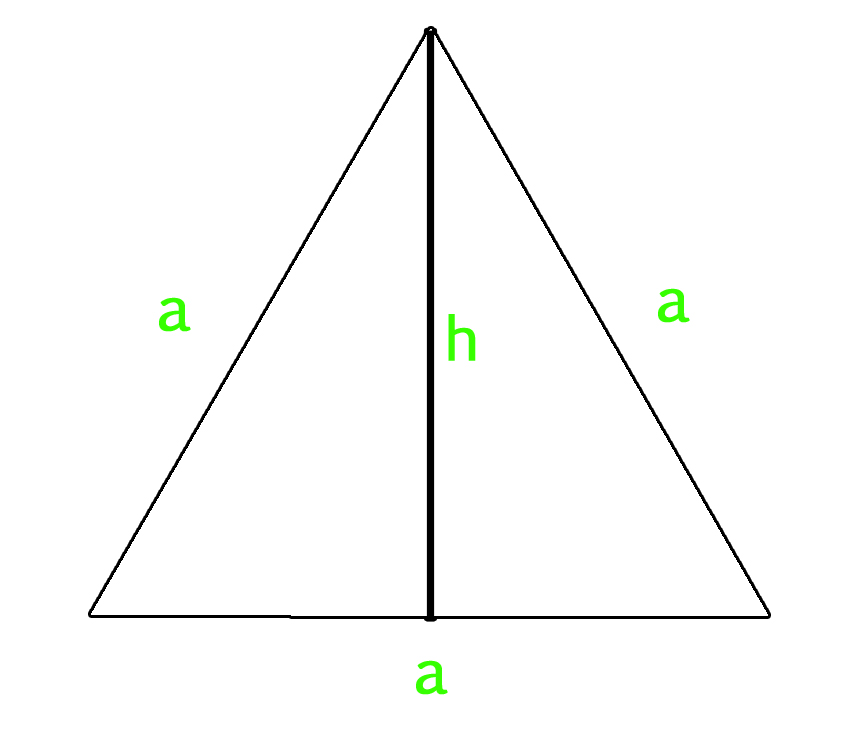Related Articles

# Program to calculate area and perimeter of equilateral triangle

• Last Updated : 18 Oct, 2019

An equilateral triangle is a triangle in which all three sides and angles are equal. All three internal angles of equilateral triangle measures 60 degree.

Area of Equilateral triangle :

• If we know the length of each sides of equilateral triangle, then we can use below mentioned formula to calculate area of equilateral triangle.
`Area of Equilateral Triangle = (sqrt(3)/4) * a * a    `
• If we know the length of altitude of equilateral triangle along with the length of side, then we can use below mentioned formula to calculate it’s area.
`Area of Equilateral Triangle = (1/2) x Side x Altitude`

Perimeter of Equilateral Triangle :

`Perimeter of Equilateral Triangle :  3 X a`

How does the area formula work?
Let us take a look at below diagram. We know are of a triangle is 1/2 * base * height. The value of h is sqrt(a2 – (a/2)2) = sqrt(3) * a / 2. So the area becomes 1/2 * a * (sqrt(3) * a / 2) = (sqrt(3)/4) * a * aExamples :

```Input : side = 4
Output : Area of Equilateral Triangle: 6.9282
Perimeter of Equilateral Triangle: 12

Input : side = 12
Output : Area of Equilateral Triangle: 62.3538
Perimeter of Equilateral Triangle: 36
```

## C++

 `// CPP program to find area``// and perimeter of equilateral triangle``#include ``using` `namespace` `std;`` ` `// Function to calculate Area ``// of equilateral triangle``float` `area_equi_triangle(``float` `side)``{``    ``return` `sqrt``(3) / 4 * side * side;``}`` ` `// Function to calculate Perimeter ``// of equilateral triangle``float` `peri_equi_triangle(``float` `side)``{``    ``return` `3 * side;``}`` ` `// Driver Code``int` `main()``{``    ``float` `side = 4;``    ``cout << ``"Area of Equilateral Triangle: "``         ``<< area_equi_triangle(side) << endl;``    ``cout << ``"Perimeter of Equilateral Triangle: "``         ``<< peri_equi_triangle(side);``    ``return` `0;``}`

## Java

 `// Java Program to find area and``// perimeter of equilateral triangle``import` `java.io.*;`` ` `class` `GFG ``{``    ``// Function to calculate ``    ``// Area of equilateral triangle``    ``static` `float` `area_equi_triangle(``float` `side)``    ``{`` ` `        ``return` `(``float``)(((Math.sqrt(``3``)) / ``4``) *``                         ``side * side);``    ``}`` ` `    ``// Function to calculate ``    ``// Perimeter of equilateral``    ``// triangle``    ``static` `float` `peri_equi_triangle(``float` `side)``    ``{``        ``return` `3` `* side;``    ``}``     ` `    ``// Driver Code``    ``public` `static` `void` `main(String arg[])``    ``{``        ``float` `side = ``4``;``        ``System.out.print(``"Area of Equilateral Triangle:"``);``        ``System.out.println(area_equi_triangle(side));``        ``System.out.print(``"Perimeter of Equilateral Triangle:"``);``        ``System.out.println(peri_equi_triangle(side));``    ``}``}`` ` `// This code is contributed ``// by Anant Agarwal.`

## Python

 `# Python3 program to calculate Area and ``# Perimeter of equilateral Triangle`` ` `# Importing Math library for sqrt``from` `math ``import` `*`` ` `# Function to calculate Area ``# of equilateral triangle``def` `area_equilateral( side ):``    ``area ``=` `(sqrt(``3``) ``/` `4``) ``*` `side ``*` `side``    ``print` `(``"Area of Equilateral Triangle: % f"``%` `area)`` ` `# Function to calculate Perimeter``# of equilateral triangle ``def` `perimeter( side ):``    ``perimeter ``=` `3` `*` `side``    ``print` `(``"Perimeter of Equilateral Triangle: % f"``%` `perimeter)``     ` `# Driver code``side ``=` `4``area_equilateral( side )``perimeter( side )`

## C#

 `// C# Program to find area and``// perimeter of equilateral triangle``using` `System;`` ` `class` `GFG ``{``    ``// Function to calculate ``    ``// Area of equilateral triangle``    ``static` `float` `area_equi_triangle(``float` `side)``    ``{`` ` `        ``return` `(``float``)(((Math.Sqrt(3)) / 4) * ``                         ``side * side);``    ``}`` ` `    ``// Function to calculate ``    ``// Perimeter of equilateral``    ``// triangle``    ``static` `float` `peri_equi_triangle(``float` `side)``    ``{``        ``return` `3 * side;``    ``}``     ` `    ``// Driver Code``    ``public` `static` `void` `Main()``    ``{``        ``float` `side = 4;``        ``Console.Write(``"Area of Equilateral Triangle:"``);``        ``Console.WriteLine(area_equi_triangle(side));``        ``Console.Write(``"Perimeter of Equilateral Triangle:"``);``        ``Console.WriteLine(peri_equi_triangle(side));``    ``}``}`` ` `// This code is contributed ``// by vt_m.`

## PHP

 ``

Output :
```Area of Equilateral Triangle: 6.9282
Perimeter of Equilateral Triangle: 12```

Attention reader! Don’t stop learning now. Participate in the Scholorship Test for First-Step-to-DSA Course for Class 9 to 12 students.

My Personal Notes arrow_drop_up